# Ping Pong Robot V1 DIY

Interactive robot to train ping pong - V1.You will never be alone again in this life, if you, at least , have one TTR (Table Tennis Robot)

IntermediateProtip2,460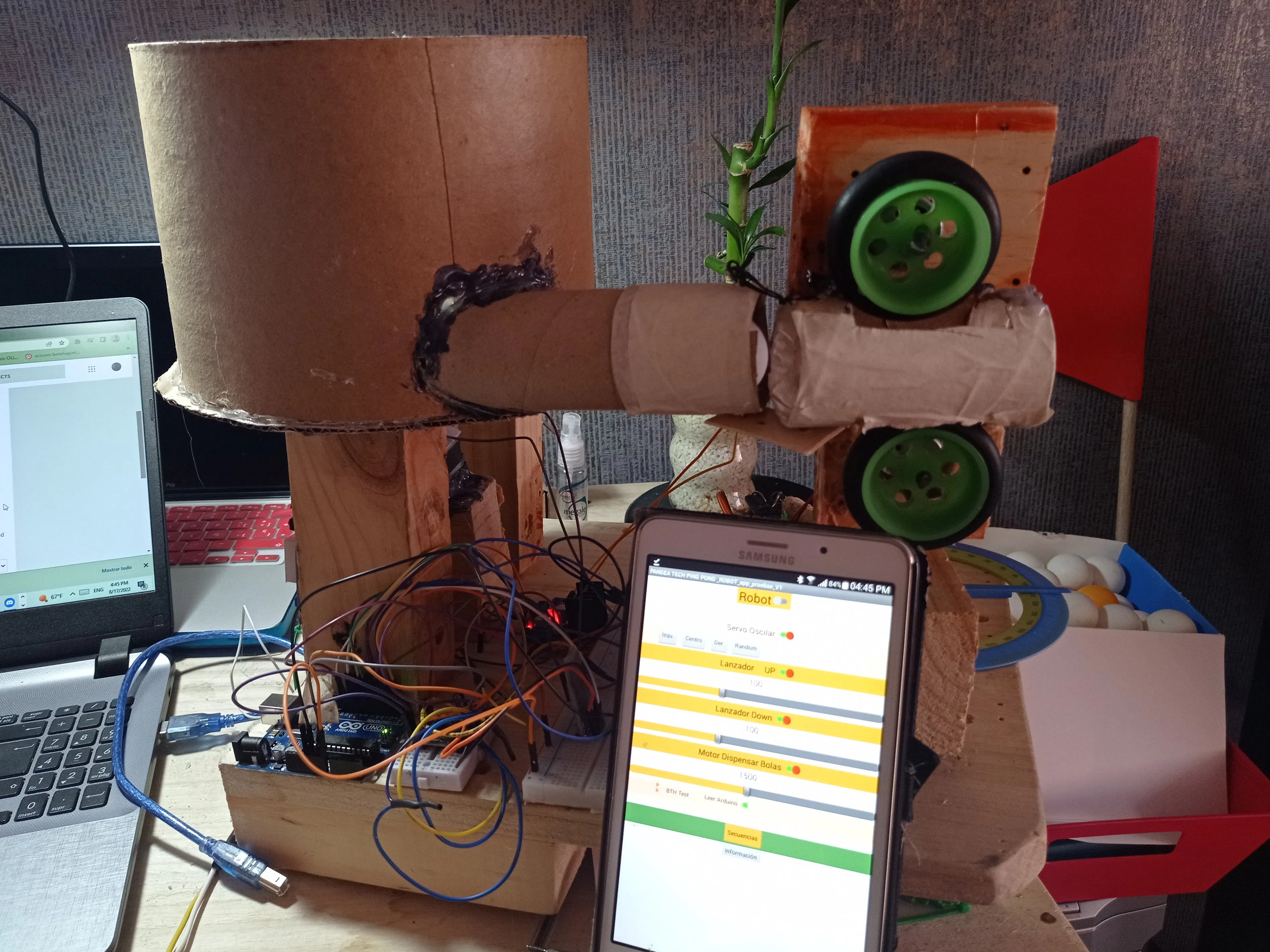## Things used in this project

### Hardware componentsArduino UNO
×1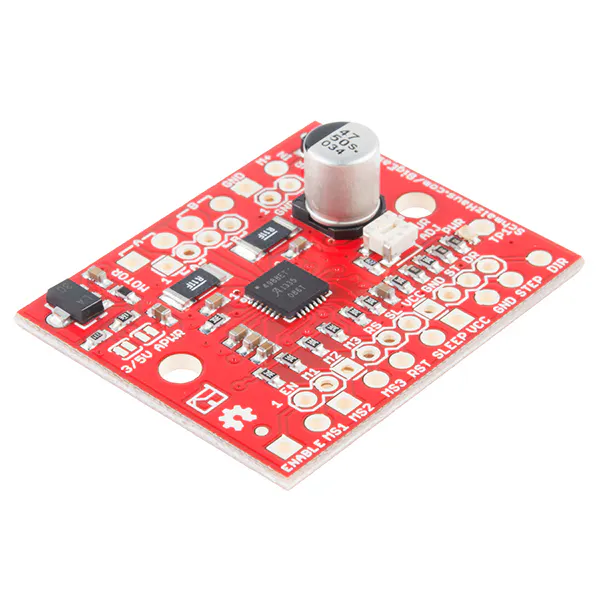SparkFun Stepper motor driver board A4988
×1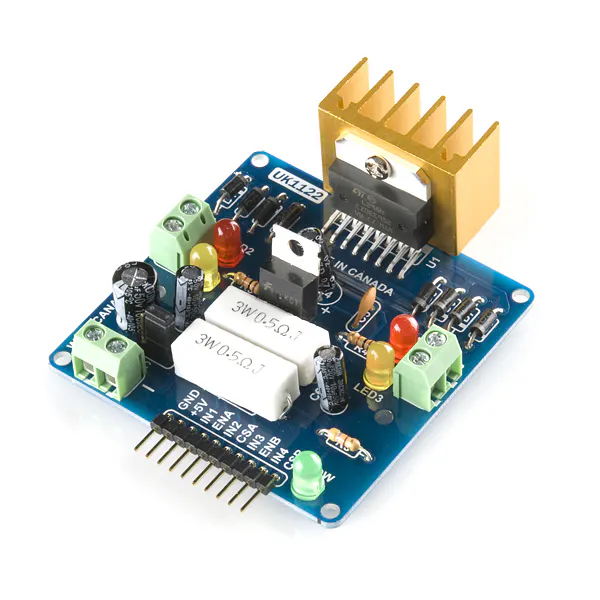SparkFun Dual H-Bridge motor drivers L298
×1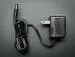9V 1A Switching Wall Power Supply
×1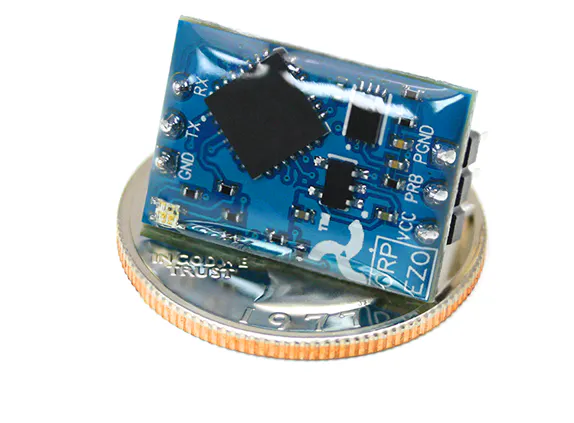Atlas Scientific EZO™ Oxidation-Reduction Potential Circuit
×1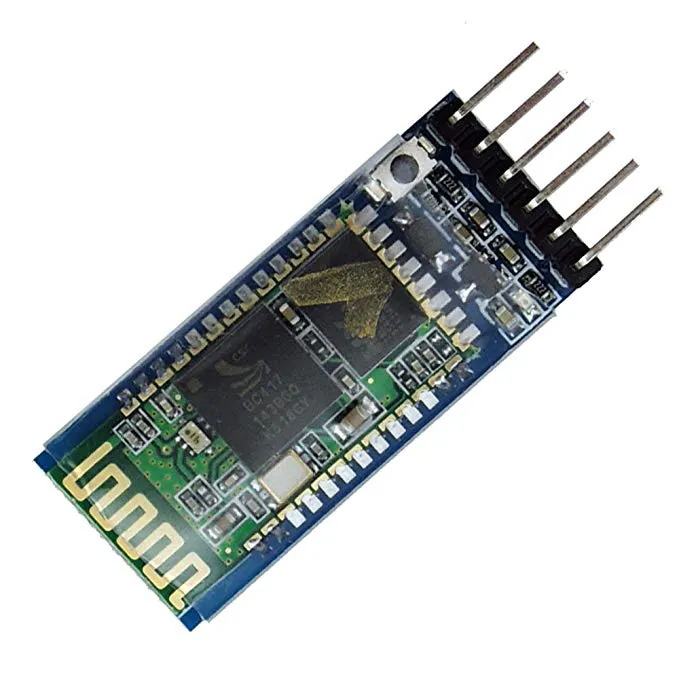HC-05 Bluetooth Module
×1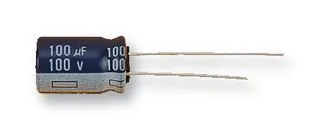Capacitor 100 µF
×1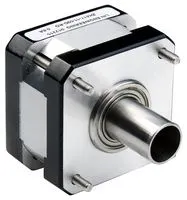Stepper Motor, Bipolar
×1×2
 TALENTEC entertainment Servo MG995
×1Jumper wires (generic)
×1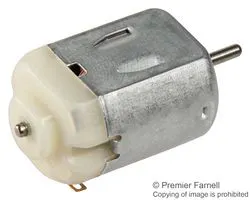DC Motor, Miniature
×1

 TTR v1

### Hand tools and fabrication machinesHot glue gun (generic)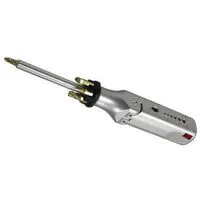Multitool, Screwdriver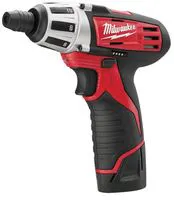Drill, Screwdriver

## Schematics

### Schematics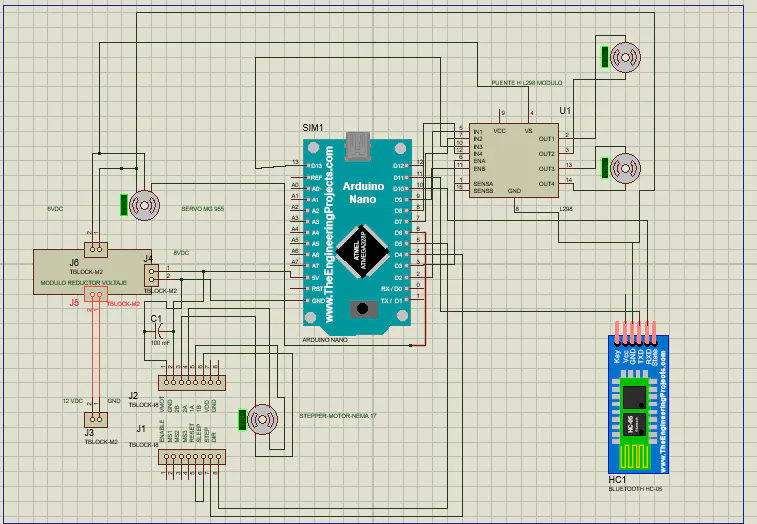## Code

### Table Tennis Robot

C/C++
This code run with the APP developed in APP inventor . The APP is the HMI only use the reset button of the arduino to restart the TTR. check the app here: https://gallery.appinventor.mit.edu/?galleryid=3f3c208a-559f-4aaa-9d3c-0d556000bcbb
```//* Ping Pong Robot Inicio de codigo: version 1- Julio 28-2022
// *  by hernando Bolaños
// *
// * 01-08-2022- servo variacion update
//* Pruebas  comunicaciones con APP
//*15-08-2022 , agregar botones de iz-centro-derecha y random - reciben pero interfieren con servo en oscilación
//*15-08-2022 -primeras pruebas de sliders- no operan correctamente con parseint() -debido a que estamos trabajando con multitasking
//

//////////////////////////////////////////////////////////////////////////////////////////////////////
////WARNING: DRAFT CODE////The robot must need adult supervision if are kids playing with it
/////////////////////////////////////////////////////////////////////////////////////////////////////
//-- Zona de librerías --
// Comunicación serie por software
#include <SoftwareSerial.h>

//-- Zona de definiciones --
#define LED
#define RX 11
#define TX 10
const int pinENA = 3; // Motor UP PWM
const int pinIN1 = 2;// motor UP
const int pinIN2 = 7; // Motor UP
const int pinENB = 9;// Motor Down PWM
const int pinIN3 = 8;//Motor Down
const int pinIN4 = 13;//Motor Down

const int speed = 250;      //velocidad de giro 80% (200/255)

const int speedoff = 0;      //velocidad de giro 80% (200/255)

long unsigned valor=0;

//-- Zona de variables globales
SoftwareSerial BT1(RX,TX);

#define STEP_L 4    // pin STEP de A4988 a pin 4manejando la cara left del cubo
#define DIR_L 5     // pin DIR de A4988 a pin 5  manejandola cara left del cubo
#define ENAB 12     // pIN ENAB
int pasos = 200;    // LATERALES pasos   90 grados con motor Hanpose 17HS3430 : 1.8 grados por paso en condicion Full step- Se probo comportamiento en otras configuraciones de pasos
int tiempo_step = 1800; //es invertido-entre menos es el valor mas rapido va el stepper a variar con HMI - microseconds -segun DS minimo tiempo permitido del step de motores 1 microsegundo

//

#include <Servo.h>

Servo myservo;  // create servo object to control a servo
int servo_angle = 90;//90
int servo_angle1 = 60;// valor para quedar en posicion central fija
int variacion = 10;/// con esta variable se puede hacer mas lenta o mas rapida la osiclacion
unsigned long startTime_servo = 0;
unsigned long interval_servo = 1100;//t1100
int latchball = 0;
int latchservo = 0;
int latchup = 0;
int latchdown =0;
int latchservoizq =0;
int latchservocen =0;
int latchservoder =0;
int latchservorandom =0;

void setup()
{

pinMode(STEP_L, OUTPUT);  // pin 4 como salida
pinMode(DIR_L, OUTPUT);   // pin 5 como salida
pinMode(ENAB, OUTPUT);  // pIN 12 como salida para driver A4998
pinMode(pinIN1, OUTPUT);
pinMode(pinIN2, OUTPUT);
pinMode(pinENA, OUTPUT);//PWM
pinMode(pinIN3, OUTPUT);
pinMode(pinIN4, OUTPUT);
pinMode(pinENB, OUTPUT);//PWM

myservo.attach(6);  // attaches the servo on pin 6 to the servo object
Serial.begin(9600);

myservo.write(90); // usado al inicio para verificar si el servo esta activo
myservo.write (0); // ir a posicion de inicio en el centro
myservo.write(60); // usado al inicio para verificar si el servo esta activo

BT1.begin(9600);
char recibido=0;
int valor=0;
int i=0;
}

void loop()
{

unsigned currentTime = millis();
char recibido=0;

long unsigned valor=0;

//Si se ha recibido un dato
if(BT1.available()==true){
recibido=BT1.read(); //Se carga en la variable recibido
Serial.print(recibido,0);
//long unsigned valor = BT1.parseInt();

//  int speed= valor;
//           Serial.print(valor);
//Serial.print(speed);

}

//

if (recibido == 'A'){

int latchrobot = 1;

}

if (recibido == 'B'){

int latchrobot = 0;

latchball = 0; //desenganchar
latchservo =0; //desenganchar
latchup=0;//desenganchar
latchdown=0;//desenganchar
latchservoizq=0;//desenganchar
latchservoder=0;//desenganchar
latchservocen=0;//desengachar
digitalWrite(ENAB, HIGH); //aplicar  Modo Disable del driver

}

if (recibido == 'C'){

latchball= 1;}

if (recibido == 'D'){

latchball= 0;}

if (recibido == 'E'){

latchup= 1;}

if (recibido == 'F'){

latchup= 0;}

if (recibido == 'G'){

latchdown= 1;}

if (recibido == 'H'){

latchdown= 0;}

if (recibido == 'I'){

latchservo = 1;}

if (recibido == 'J'){

latchservo = 0;}

if (recibido == 'Z'){

latchservoizq = 1;}

if (recibido == 'W'){

latchservoizq = 0;}

if (recibido == 'T'){

latchservocen = 1;}

if (recibido == 'X'){

latchservocen = 0;}

if (recibido == 'R'){

latchservoder = 1;}

if (recibido == 'Y'){

latchservoder = 0;}

if (recibido == 'M'){

latchservorandom = 1;}

if (recibido == 'N'){

latchservorandom = 0;}

//if (((valor >=20) and (valor <=25)) and(( latchup=1) and (latchdown =1) )){ const int speed=valor; Serial.print(speed);} // tomando trama de los slider up y down

if (latchball == 1){
alimbolas();
//BT1.write('C');

}

if (latchball  == 0){
//Serial.println("parar Dispensar Bolas activado");
//BT1.write('D');

}

if (latchservo == 1){

oscilar();
//BT1.write('I');}

}

if (latchservo == 0){

//BT1.write('J');

}

if (latchup == 1){

uprob();

}

if (latchup == 0){

upoff();
//BTI.write(' ');

}

if (latchdown == 1){

down();

}

if (latchdown == 0){

downoff();
//BTI.write(' ');

}

if (latchservoizq== 1){

//
int i=0;

for (int i = 0; i <= 1; i++) {
oscizq();
}

if (i>=1){
latchservoizq == 0;}
//Serial.println("Fijo en izquierda Activado");
//BTI.write(' ');

}

if (latchservoizq == 0){

//oscizqoff();
//Serial. println(" Fijo en ziquiera desActivado")
//BTI.write(' ');

}

if (latchservocen== 1){

int i=0;

for (int i = 0; i <= 1; i++) {
osccentro();
}

if (i>=1){
latchservocen == 0;}
//Serial. println("Fijo en centro Activado");
//BTI.write(' ');

}

if (latchservocen == 0){

//osccentrooff();
//Serial. println(" Fijo en centro desaActivado)
//BTI.write(' ');

}

if (latchservoder== 1){

int i=0;

for (int i = 0; i <= 1; i++) {
oscder();
}
if (i>=1){
latchservoder == 0;}
//Serial.println("Fijo en centro Activado");
//BTI.write(' ');

}

if (latchservoder == 0){

//oscderoff();

//Serial. println(" Fijo en centro desaActivado)
//BTI.write(' ');

}
}

void alimbolas()// alimenta bolas giando en sentido NCW

{

Serial.print("Bolas NCW,");

digitalWrite(ENAB, LOW); // Modo Enable del driver

digitalWrite(DIR_L, HIGH);    // giro en  sentido NCW
for (int i = 0; i < pasos; i++) {   //
digitalWrite(STEP_L, HIGH);       // nivel alto
delayMicroseconds(tiempo_step);          // por x mseg
delayMicroseconds(tiempo_step);
digitalWrite(STEP_L, LOW);        // nivel bajo
delayMicroseconds(tiempo_step);          // por x mseg
delayMicroseconds(tiempo_step);
}

}

void oscilar() //activa modo  oscilar en el robot - izquierda a derecha
{
unsigned currentTime = millis();

//Serial.println("servo");
if (currentTime - startTime_servo >= interval_servo){
startTime_servo=currentTime;
myservo.write(servo_angle);
servo_angle = servo_angle + variacion;
if (servo_angle == 160) servo_angle = 30;
//Serial.println(servo_angle);
}
}

void oscizq() //activa modo  oscilar en el robot - izquierda a derecha
{
unsigned currentTime = millis();

//Serial.println("servo");
if (currentTime - startTime_servo >= interval_servo){
startTime_servo=currentTime;
myservo.write(160);

//Serial.println(servo_angle);
}
}

void oscder() //activa modo  oscilar en el robot - izquierda a derecha
{
unsigned currentTime = millis();

//Serial.println("servo");
if (currentTime - startTime_servo >= interval_servo){
startTime_servo=currentTime;
myservo.write(0);

}
}

void osccentro() //activa modo  oscilar en el robot - izquierda a derecha
{
unsigned currentTime = millis();

//Serial.println("servo");
if (currentTime - startTime_servo >= interval_servo){
startTime_servo=currentTime;
myservo.write(90);

}
}

void oscizqoff() //activa modo  oscilar en el robot - izquierda a derecha
{
unsigned currentTime = millis();

//Serial.println("servo");
if (currentTime - startTime_servo >= interval_servo){
startTime_servo=currentTime;
myservo.write(90);

//Serial.println(servo_angle);
}
}

void oscderoff() //activa modo  oscilar en el robot - izquierda a derecha
{
unsigned currentTime = millis();

//Serial.println("servo");
if (currentTime - startTime_servo >= interval_servo){
startTime_servo=currentTime;
myservo.write(90);

}
}

void osccentrooff() //activa modo  oscilar en el robot - izquierda a derecha
{
unsigned currentTime = millis();

//Serial.println("servo");
if (currentTime - startTime_servo >= interval_servo){
startTime_servo=currentTime;
myservo.write(90);

}
}

void uprob()  //
{
//Serial.println("corriendo rutina UP,");
//   Serial.println(speed);
digitalWrite(pinIN1, HIGH);
digitalWrite(pinIN2, LOW);
analogWrite(pinENA, speed);
//delay(1000);
}

void upoff()
{ //Serial.println("corriendo rutina upoff");

digitalWrite(pinIN1, LOW);
digitalWrite(pinIN2, LOW);
analogWrite(pinENA, speedoff);
//delay(1000);
}

void down()
{
digitalWrite(pinIN3, LOW);
digitalWrite(pinIN4, HIGH);
analogWrite(pinENB, speed);
//delay(1000);
}

void downoff()
{
digitalWrite(pinIN3, LOW);
digitalWrite(pinIN4, LOW);
analogWrite(pinENB, speedoff);
//delay(1000);
}
```

## Credits

### hbolanos2001

9 projects • 28 followers